Home
Hostname: page-component-7ccbd9845f-4v6tc Total loading time: 0.487 Render date: 2023-01-28T01:33:48.367Z Has data issue: true Feature Flags: { "useRatesEcommerce": false } hasContentIssue true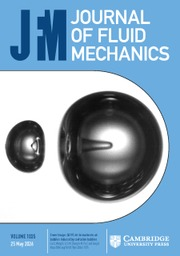Journal of Fluid Mechanics

## Abstract

Drag control using a newly developed spanwise opposed wall-jet forcing (SOJF) method is studied via direct numerical simulation of the incompressible Navier–Stokes equations in a turbulent channel flow (at the friction Reynolds numbers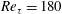$Re_{\unicode[STIX]{x1D70F}}=180$ and 550). SOJF is characterized by three control parameters: the forcing amplitude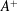$A^{+}$, the spanwise spacing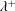$\unicode[STIX]{x1D706}^{+}$ and the wall-jet height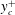$y_{c}^{+}$ (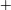$+$ indicates viscous scaling). At$Re_{\unicode[STIX]{x1D70F}}=180$, notable drag reduction is achieved for wide ranges of$A^{+}$,$\unicode[STIX]{x1D706}^{+}$ and$y_{c}^{+}$, with an optimal drag reduction of approximately 19 % found for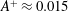$A^{+}\approx 0.015$,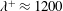$\unicode[STIX]{x1D706}^{+}\approx 1200$ and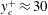$y_{c}^{+}\approx 30$. The drag reduction results from mergers of numerous low-speed typical individual streaks together by the wall jets, so that the slope of the merged streak envelope and hence the streak strength are reduced below the critical values required for streak instability as well as for transient growth; consequently, the generation of drag inducing near-wall streamwise vortices is suppressed. Through analysis using the FIK identity (Fukagata et al. Phys. Fluids, vol. 14 (11), 2002, pp. L73–L76) in combination with the triple decomposition and the spanwise wavenumber spectrum of the Reynolds shear stress, we find that the control significantly decreases skin friction due to the small scale random turbulent structures (from 75 to 23 % for the optimal case), but injects a dominant contribution at the forcing scale (approximately 34 %). As$A^{+}$ or$y_{c}^{+}$ increases, the drag reduction degrades due to the downwash near the initiation of the forcing wall jet. The energy input required for the excitation is found to be small, yielding a 17 % net power saving for the optimal control case. To determine the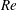$Re$ dependence of the drag reduction, the control strategy is further validated at a higher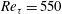$Re_{\unicode[STIX]{x1D70F}}=550$. If the control parameters are kept the same as at$Re_{\unicode[STIX]{x1D70F}}=180$ (i.e.$A^{+}\approx 0.015$,$\unicode[STIX]{x1D706}^{+}\approx 1200$,$y_{c}^{+}\approx 30$), the drag reduction decreases to 10 %; however, interestingly, with modestly changed parameters (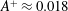$A^{+}\approx 0.018$,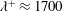$\unicode[STIX]{x1D706}^{+}\approx 1700$,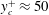$y_{c}^{+}\approx 50$), drag reduction increases to about 15 %. This additional drag reduction results from the further suppression of turbulent structures in the buffer and log regions. This result, therefore, suggests prospects for drag reduction at even higher$Re$ via a proper choice of the SOJF parameters.

## JFM classification

Type
JFM Papers
Information
Journal of Fluid Mechanics , 10 October 2018 , pp. 678 - 709

## Access options

Get access to the full version of this content by using one of the access options below. (Log in options will check for institutional or personal access. Content may require purchase if you do not have access.)

## References

Abbassi, M. R., Baars, W. J., Hutchins, N. & Marusic, I. 2017 Skin-friction drag reduction in a high-Reynolds-number turbulent boundary layer via real-time control of large-scale structures. Intl J. Heat Fluid Flow 67, 3041.CrossRefGoogle Scholar
Agostini, L., Touber, E. & Leschziner, M. A. 2014 Spanwise oscillatory wall motion in channel flow: drag-reduction mechanisms inferred from dns-predicted phase-wise property variations at ret = 1000. J. Fluid Mech. 743, 606635.CrossRefGoogle Scholar
Akhavan, R., Jung, W. J. & Mangiavacchi, N. 1993 Turbulence control in wall-bounded flows by spanwise oscillations. In Advances in Turbulence IV, pp. 299303. Springer.CrossRefGoogle Scholar
Bechert, D. W., Bruse, M., Hage, W., Van der Hoeven, J. G. T. & Hoppe, G. 1997 Experiments on drag-reducing surfaces and their optimization with an adjustable geometry. J. Fluid Mech. 338, 5987.CrossRefGoogle Scholar
Berger, T. W., Kim, J., Lee, C. & Lim, J. 2000 Turbulent boundary layer control utilizing the lorentz force. Phys. Fluids 12 (3), 631649.CrossRefGoogle Scholar
Butler, K. M. & Farrell, B. F. 1993 Optimal perturbations and streak spacing in wall-bounded turbulent shear flow. Phys. Fluids A 5 (3), 774777.CrossRefGoogle Scholar
Canton, J., Örlü, R., Chin, C., Hutchins, N., Monty, J. & Schlatter, P. 2016a On large-scale friction control in turbulent wall flow in low Reynolds number channels. Flow Turbul. Combust. 97, 117.CrossRefGoogle Scholar
Canton, J., Örlü, R., Chin, C. & Schlatter, P. 2016b Reynolds number dependence of large-scale friction control in turbulent channel flow. Phys. Rev. Fluids 1 (8), 081501.CrossRefGoogle Scholar
Checco, A., Ocko, B. M., Rahman, A., Black, C. T., Tasinkevych, M., Giacomello, A. & Dietrich, S. 2014 Collapse and reversibility of the superhydrophobic state on nanotextured surfaces. Phys. Rev. Lett. 112 (21), 216101.CrossRefGoogle Scholar
Choi, H., Moin, P. & Kim, J. 1994 Active turbulence control for drag reduction in wall-bounded flows. J. Fluid Mech. 262, 75110.CrossRefGoogle Scholar
Choi, J.-I., Xu, C.-X. & Sung, H. J. 2002 Drag reduction by spanwise wall oscillation in wall-bounded turbulent flows. AIAA J. 40 (5), 842850.CrossRefGoogle Scholar
Chung, Y. M. & Talha, T. 2011 Effectiveness of active flow control for turbulent skin friction drag reduction. Phys. Fluids 23 (2), 025102.CrossRefGoogle Scholar
Corke, T. C., Enloe, C. L. & Wilkinson, S. P. 2010 Dielectric barrier discharge plasma actuators for flow control. Annu. Rev. Fluid Mech. 42, 505529.CrossRefGoogle Scholar
Deng, B.-Q. & Xu, C.-X. 2012 Influence of active control on stg-based generation of streamwise vortices in near-wall turbulence. J. Fluid Mech. 710, 234259.CrossRefGoogle Scholar
Duvvuri, S. & McKeon, B. J. 2015 Triadic scale interactions in a turbulent boundary layer. J. Fluid Mech. 767, R4.CrossRefGoogle Scholar
Erfani, R., Zare-Behtash, H., Hale, C. & Kontis, K. 2015 Development of dbd plasma actuators: the double encapsulated electrode. Acta Astron. 109, 132143.CrossRefGoogle Scholar
Fukagata, K., Iwamoto, K. & Kasagi, N. 2002 Contribution of Reynolds stress distribution to the skin friction in wall-bounded flows. Phys. Fluids 14 (11), L73L76.CrossRefGoogle Scholar
Gatti, D. & Quadrio, M. 2013 Performance losses of drag-reducing spanwise forcing at moderate values of the Reynolds number. Phys. Fluids 25 (12), 125109.CrossRefGoogle Scholar
Gatti, D. & Quadrio, M. 2016 Reynolds-number dependence of turbulent skin-friction drag reduction induced by spanwise forcing. J. Fluid Mech. 802, 553582.CrossRefGoogle Scholar
Ghebali, S., Chernyshenko, S. I. & Leschziner, M. A. 2017 Can large-scale oblique undulations on a solid wall reduce the turbulent drag? Phys. Fluids 29 (10), 105102.CrossRefGoogle Scholar
de Giovanetti, M., Hwang, Y. & Choi, H. 2016 Skin-friction generation by attached eddies in turbulent channel flow. J. Fluid Mech. 808, 511538.CrossRefGoogle Scholar
de Giovanetti, M., Sung, H. J. & Hwang, Y. 2017 Streak instability in turbulent channel flow: the seeding mechanism of large-scale motions. J. Fluid Mech. 832, 483513.CrossRefGoogle Scholar
Hamilton, J. M., Kim, J. & Waleffe, F. 1995 Regeneration mechanisms of near-wall turbulence structures. J. Fluid Mech. 287, 317348.CrossRefGoogle Scholar
Iuso, G., Onorato, M., Spazzini, P. G. & Di Cicca, G. M. 2002 Wall turbulence manipulation by large-scale streamwise vortices. J. Fluid Mech. 473, 2358.CrossRefGoogle Scholar
Jeong, J. & Hussain, F. 1995 On the identification of a vortex. J. Fluid Mech. 285, 6994.CrossRefGoogle Scholar
Jiménez, J. & Pinelli, A. 1999 The autonomous cycle of near-wall turbulence. J. Fluid Mech. 389, 335359.CrossRefGoogle Scholar
Jung, W. J., Mangiavacchi, N. & Akhavan, R. 1992 Suppression of turbulence in wall-bounded flows by high-frequency spanwise oscillations. Phys. Fluids A 4 (8), 16051607.CrossRefGoogle Scholar
Kim, J. 2011 Physics and control of wall turbulence for drag reduction. Phil. Trans. R. Soc. Lond. A 369 (1940), 13961411.CrossRefGoogle ScholarPubMed
Kim, J. & Bewley, T. R. 2007 A linear systems approach to flow control. Annu. Rev. Fluid Mech. 39, 383417.CrossRefGoogle Scholar
Kline, S. J., Reynolds, W. C., Schraub, F. A. & Runstadler, P. W. 1967 The structure of turbulent boundary layers. J. Fluid Mech. 30 (04), 741773.CrossRefGoogle Scholar
Laizet, S. & Lamballais, E. 2009 High-order compact schemes for incompressible flows: a simple and efficient method with quasi-spectral accuracy. J. Comput. Phys. 228 (16), 59896015.CrossRefGoogle Scholar
Laizet, S. & Li, N. 2011 Incompact3d: a powerful tool to tackle turbulence problems with up to O (105) computational cores. Intl J. Numer. Meth. Fluids 67 (11), 17351757.CrossRefGoogle Scholar
Lee, M. & Moser, R. D. 2015 Direct numerical simulation of turbulent channel flow up to Re 5200. J. Fluid Mech. 774, 395415.CrossRefGoogle Scholar
Li, Y. P., Wong, C. W., Li, Y. Z., Zhang, B. F. & Zhou, Y. 2014 Drag reduction of a turbulent boundary layer using plasma actuators. In Proceeding of the 19th Australasian Fluid Mechanics Conference, Australasian Fluid Mechanics Society.Google Scholar
Mahfoze, O. & Laizet, S. 2017 Skin-friction drag reduction in a channel flow with streamwise-aligned plasma actuators. Intl J. Heat Fluid Flow 66, 8394.CrossRefGoogle Scholar
Mamori, H., Iwamoto, K. & Murata, A. 2014 Effect of the parameters of traveling waves created by blowing and suction on the relaminarization phenomena in fully developed turbulent channel flow. Phys. Fluids 26 (1), 015101.CrossRefGoogle Scholar
Oliver, T. A., Malaya, N., Ulerich, R. & Moser, R. D. 2014 Estimating uncertainties in statistics computed from direct numerical simulation. Phys. Fluids 26 (3), 035101.CrossRefGoogle Scholar
Park, H., Sun, G. & Kim, C.-J. 2014 Superhydrophobic turbulent drag reduction as a function of surface grating parameters. J. Fluid Mech. 747, 722734.CrossRefGoogle Scholar
Quadrio, M. 2011 Drag reduction in turbulent boundary layers by in-plane wall motion. Phil. Trans. R. Soc. Lond. A 369 (1940), 14281442.CrossRefGoogle ScholarPubMed
Quadrio, M. & Ricco, P. 2004 Critical assessment of turbulent drag reduction through spanwise wall oscillations. J. Fluid Mech. 521, 251271.CrossRefGoogle Scholar
Quadrio, M., Ricco, P. & Viotti, C. 2009 Streamwise-travelling waves of spanwise wall velocity for turbulent drag reduction. J. Fluid Mech. 627, 161178.CrossRefGoogle Scholar
Rastegari, A. & Akhavan, R. 2015 On the mechanism of turbulent drag reduction with super-hydrophobic surfaces. J. Fluid Mech. 773, R4.CrossRefGoogle Scholar
Rastegari, A. & Akhavan, R. 2018 The common mechanism of turbulent skin-friction drag reduction with superhydrophobic longitudinal microgrooves and riblets. J. Fluid Mech. 838, 68104.CrossRefGoogle Scholar
Reynolds, W. C. & Hussain, A. K. M. F. 1972 The mechanics of an organized wave in turbulent shear flow. Part 3. Theoretical models and comparisons with experiments. J. Fluid Mech. 54 (2), 263288.CrossRefGoogle Scholar
Ricco, P. & Hahn, S. 2013 Turbulent drag reduction through rotating discs. J. Fluid Mech. 722, 267290.CrossRefGoogle Scholar
Ricco, P. & Quadrio, M. 2008 Wall-oscillation conditions for drag reduction in turbulent channel flow. Intl J. Heat Fluid Flow 29 (4), 891902.CrossRefGoogle Scholar
Rothstein, J. P. 2010 Slip on superhydrophobic surfaces. Annu. Rev. Fluid Mech. 42, 89109.CrossRefGoogle Scholar
Schlatter, P., Li, Q., Örlü, R., Hussain, F. & Henningson, D. S. 2014 On the near-wall vortical structures at moderate Reynolds numbers. Eur. J. Mech. (B/Fluids) 48, 7593.CrossRefGoogle Scholar
Schoppa, W. & Hussain, F. 1998 A large-scale control strategy for drag reduction in turbulent boundary layers. Phys. Fluids 10 (5), 10491051.CrossRefGoogle Scholar
Schoppa, W. & Hussain, F. 2002 Coherent structure generation in near-wall turbulence. J. Fluid Mech. 453, 57108.CrossRefGoogle Scholar
Segawa, T., Mizunuma, H., Murakami, K., Li, F.-C. & Yoshida, H. 2007 Turbulent drag reduction by means of alternating suction and blowing jets. Fluid Dyn. Res. 39 (7), 552568.CrossRefGoogle Scholar
Smith, C. R. & Metzler, S. P. 1983 The characteristics of low-speed streaks in the near-wall region of a turbulent boundary layer. J. Fluid Mech. 129, 2754.CrossRefGoogle Scholar
Thomas, F., Corke, T., Hussain, F., Duong, A., McGowan, R., Jasinski, C. & Simmons, D. 2016 Turbulent boundary layer drag reduction by active control of streak transient growth. In Bulletin of the APS, 69th Annual Metting of the APS Division of Fluid Dynamics, Vol. 61, No. 20, American Physics Society.Google Scholar
Touber, E. & Leschziner, M. A. 2012 Near-wall streak modification by spanwise oscillatory wall motion and drag-reduction mechanisms. J. Fluid Mech. 693, 150200.CrossRefGoogle Scholar
Willis, A. P., Hwang, Y. & Cossu, C. 2010 Optimally amplified large-scale streaks and drag reduction in turbulent pipe flow. Phys. Rev. E 82 (3), 036321.Google ScholarPubMed
Xi, C., Hussain, F. & She, Z.-S. 2018 Quantifying wall turbulence via a symmetry approach. Part 2. Reynolds stresses. J. Fluid Mech. 850, 401438.Google Scholar
Yakeno, A., Hasegawa, Y. & Kasagi, N. 2014 Modification of quasi-streamwise vortical structure in a drag-reduced turbulent channel flow with spanwise wall oscillation. Phys. Fluids 26 (8), 085109.CrossRefGoogle Scholar
Yao, J., Chen, X., Thomas, F. & Hussain, F. 2017 Large-scale control strategy for drag reduction in turbulent channel flows. Phys. Rev. Fluids 2, 062601(R).CrossRefGoogle Scholar
33
Cited by

# Save article to Kindle

Note you can select to save to either the @free.kindle.com or @kindle.com variations. ‘@free.kindle.com’ emails are free but can only be saved to your device when it is connected to wi-fi. ‘@kindle.com’ emails can be delivered even when you are not connected to wi-fi, but note that service fees apply.

Find out more about the Kindle Personal Document Service.

Drag control in wall-bounded turbulent flows via spanwise opposed wall-jet forcing
Available formats
×

# Save article to Dropbox

To save this article to your Dropbox account, please select one or more formats and confirm that you agree to abide by our usage policies. If this is the first time you used this feature, you will be asked to authorise Cambridge Core to connect with your Dropbox account. Find out more about saving content to Dropbox.

Drag control in wall-bounded turbulent flows via spanwise opposed wall-jet forcing
Available formats
×

# Save article to Google Drive

To save this article to your Google Drive account, please select one or more formats and confirm that you agree to abide by our usage policies. If this is the first time you used this feature, you will be asked to authorise Cambridge Core to connect with your Google Drive account. Find out more about saving content to Google Drive.

Drag control in wall-bounded turbulent flows via spanwise opposed wall-jet forcing
Available formats
×
×